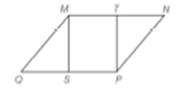Chapter 4.2, Problem 24EElementary Geometry For College St...

7th Edition
Alexander + 2 others
ISBN: 9781337614085

Solutions

Chapter
SectionElementary Geometry For College St...

7th Edition
Alexander + 2 others
ISBN: 9781337614085
Textbook Problem

In Exercise 19 to 24 , complete each proof. Given: ▱   M N P Q , with T the midpoint of M N ¯ and S the midpoint of Q P ¯ Prove: ∆ Q M S ≅ ∆ N P T and M S P T is a ▱To determine

To prove:

QMSNPT and MSPT is a

Explanation

Given:

MNPQ, with T the midpoint of MN ¯ and S the midpoint of QP¯

 PROOF Statements Reasons 1. ▱MNPQ, with T the midpoint of MN¯ and Sthe midpoint of QP¯ 1. Given 2. MN¯≅PQ¯, andNP¯≅QM¯ 2. Pairs of opposite sides of a parallelogramare congruent 3. ∠MQS≅∠PNT 3

Still sussing out bartleby?

Check out a sample textbook solution.

See a sample solution

The Solution to Your Study Problems

Bartleby provides explanations to thousands of textbook problems written by our experts, many with advanced degrees!

Get Started

In Exercises 2336, find the domain of the function. 31. f(x)=xx21

Applied Calculus for the Managerial, Life, and Social Sciences: A Brief Approach

Convert the expressions in Exercises 6584 to power form. 3x453x+43xx

Finite Mathematics and Applied Calculus (MindTap Course List)

Differentiate. f(x) = x2 sin x

Calculus: Early Transcendentals

The normal plane to at t = 1 has equation:

Study Guide for Stewart's Multivariable Calculus, 8th